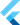# Using Tweens and Intervals

on Friday, 24th of July, 2020

Back on track, time to make those bars dance. First, lets add the AnimationController and Tween objects to the app.

``````class PegProgressIndicator extends StatefulWidget {
@override
_PegProgressIndicatorState createState() => _PegProgressIndicatorState();
}

class _PegProgressIndicatorState extends State<PegProgressIndicator> with TickerProviderStateMixin {
AnimationController _controller;
Tween<double> tween;

@override
initState() {
super.initState();
_controller = AnimationController(
duration: const Duration(milliseconds: 3000),
vsync: this,
);
tween = Tween<double>(begin: 0.0, end: 1.00);
_controller.repeat().orCancel;
}

@override
dispose() {
_controller?.dispose();
super.dispose();
}

@override
Widget build(BuildContext context) {
return  Container(
child: Center(
child: Row(
mainAxisAlignment: MainAxisAlignment.center,
children: <Widget>[
Peg()
Peg()
Peg()
Peg()
],
),
),
);
}
}``````

### Adding Tweens and Intervals

The main challenge of this animation is that it requires 8 separate steps to the overall animation, controlled by one AnimationController, distributed over only 4 widgets.

There are 8 steps in this animation because each of the four bars makes a 180degree pivot twice, and by the end of the animation they've all turned a full turn.

Luckily, Flutter also provides a way to make animations that only occur during certain times of a `Tween`. It's called an Interval.

You're going to have to write an Interval for each of the eight steps. Here's the explanation of one:

``````class _PegProgressIndicatorState extends State<PegProgressIndicator>
with TickerProviderStateMixin {
AnimationController _controller;
Tween<double> tween;

@override
initState() {
super.initState();
_controller = new AnimationController(
duration: const Duration(milliseconds: 3000),
vsync: this,
);
tween = new Tween<double>(begin: 0.0, end: 1.00);
_controller.repeat().orCancel;
}

@override
dispose() {
_controller?.dispose();
super.dispose();
}

// START NEW
// Animations always start with tweens, but you can reuse tweens.
Animation<double> get stepOne => tween.animate(
// For intervals, you can pass in an Animation rather than
// the controller
new CurvedAnimation(
// But pass in the controller here
parent: _controller,
// The interval is basically what point of the tween
// to start at, and what point to end at
// this tween is 0 to 1,
// so step one should only animate the first 1/8 of the tween
// which is 0 to 0.125
curve: new Interval(
0.0,
0.125,
// the style curve to pass.
curve: Curves.linear,
),
),
);
// ...``````

Once you've written all 8 intervals, you're going to need the widgets that actually animate during each of these 8 steps. Below you can find all 8 intervals for convenient copy and pasting.

``````  Animation<double> get stepOne => tween.animate(
CurvedAnimation(
parent: _controller,
curve: Interval(
0.0,
0.125,
curve: Curves.linear,
),
),
);
Animation<double> get stepTwo => tween.animate(
CurvedAnimation(
parent: _controller,
curve: Interval(
0.125,
0.26,
curve: Curves.linear,
),
),
);
Animation<double> get stepThree => tween.animate(
CurvedAnimation(
parent: _controller,
curve: Interval(
0.25,
0.375,
curve: Curves.linear,
),
),
);
Animation<double> get stepFour => tween.animate(
CurvedAnimation(
parent: _controller,
curve: Interval(
0.375,
0.5,
curve: Curves.linear,
),
),
);
Animation<double> get stepFive => tween.animate(
CurvedAnimation(
parent: _controller,
curve: Interval(
0.5,
0.625,
curve: Curves.linear,
),
),
);
Animation<double> get stepSix => tween.animate(
CurvedAnimation(
parent: _controller,
curve: Interval(
0.625,
0.75,
curve: Curves.linear,
),
),
);
Animation<double> get stepSeven => tween.animate(
CurvedAnimation(
parent: _controller,
curve: Interval(
0.75,
0.875,
curve: Curves.linear,
),
),
);
Animation<double> get stepEight => tween.animate(
CurvedAnimation(
parent: _controller,
curve: Interval(
0.875,
1.0,
curve: Curves.linear,
),
),
);``````